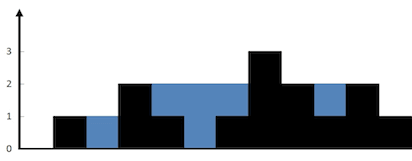Trapping Rain Water

## Description

Given n non-negative integers representing an elevation map where the width of each bar is 1, compute how >much water it is able to trap after raining.

For example, Given `[0,1,0,2,1,0,1,3,2,1,2,1]`, return `6`.The above elevation map is represented by array [0,1,0,2,1,0,1,3,2,1,2,1]. In this case, 6 units of rain water (blue section) are being trapped. Thanks Marcos for contributing this image!

## Solution

 `````` 1 2 3 4 5 6 7 8 9 10 11 12 13 14 15 16 17 18 19 20 21 22 23 24 25 26 27 28 29 30 31 32 33 34 35 36 37 38 39 40 41 42 43 44 45 46 47 `````` ``````func trap(height []int) int { var record int var result int length := len(height) if length < 3 { return 0 } leftHeight := make([]int, length) record = height // record the max height of left positions // scan left height for each position for i := 0; i < length-1; i++ { leftHeight[i] = record n := height[i] if n > record { record = n } } record = height[length-1] // record the max height of right positions for i := length - 2; i >= 0; i-- { h := min(record, leftHeight[i]) n := height[i] if h > n { // have some water result += (h - n) } if n > record { record = n } } return result } func min(a, b int) int { if a < b { return a } return b }``````

## Similar Problem

11. Container With Most Water